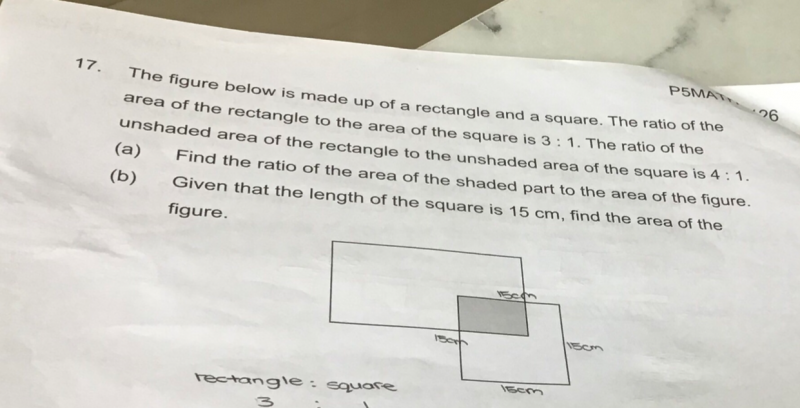# QuestionPls help to solve. Thanks.

(a) Let p be the area of the shaded part.

Area of square = u

Area of rectangle = 3u

(3u- p) / (u-p)     =     4/1

3u-p = 4u-4p

3p = u => p = u/3

Therefore, Fraction of shaded part / Total figure

=                   u/3

————————    where area of rect = 3u,  area of unshaded square = (2/3) u

3u + (2/3)u

=    (u/3) / (11u/3)

= (u/3) x (3/11u)

= 1/11

(b)  Side of square = 15cm  => Area of square = 15 x 15 = 225

Area of square = 3 out of 11 parts

Hence area of total figure =  225/3 x 11 =  825 cm2

or

u -> 225

Hence area of total figure = Area of rectangle – shaded part + Area of square

= (3u- u/3) + 225 = (3(225)-225/3) + 225 = 825 cm2